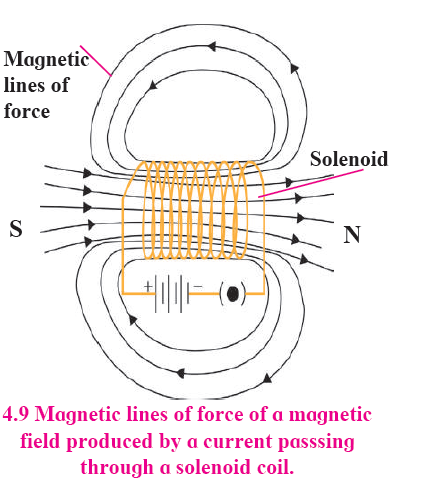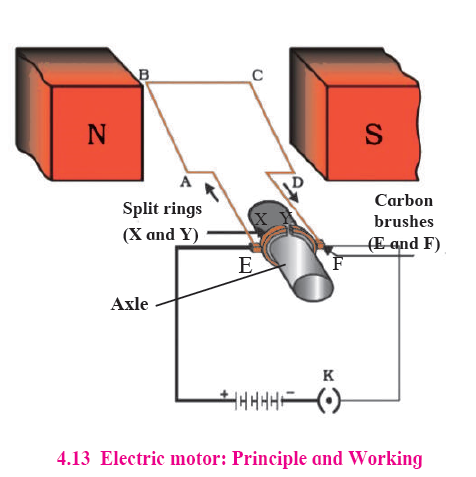# MSBSHSE Solutions For SSC (Class 10) Science Part 1 Chapter 4 - Effects of Electric Current

MSBSHSE Solutions For SSC (Class 10) Science Part 1 Chapter 4 Effects of electric current is a crucial resource that helps students to master the key concepts from the chapter thoroughly. Students can solve these and prepare efficiently for the board exam. Maharashtra Board Solutions for Class 10, also helps to promote smooth understanding of the concepts that are being covered.

This chapter mainly discusses the concepts of Effects of electric current such as Energy transfer in an electric circuit, Heating effects of electric current, Magnetic effects of electric current and more. In this chapter, students can also learn about an electric current flowing through a conducting wire, an electric current flowing through a resistor, electromagnetic induction, electric motor and generator and so on.

These solutions of MSBSHSE for Class 10 (SSC) with step-by-step explanations of the exercises given in the Maharashtra Board Science Textbooks for SSC Part 1 is also very easy to understand. The Maharashtra State Board Solutions for Chapter 4 Science Part 1 can be obtained by students. These solutions will help them if they want to revise all the key topics for the exam.

## Maharashtra Board SSC (Class 10) Science Part 1 Chapter 4- BYJU’S Important Questions & Answers

1. Tungsten metal is used to make a solenoid type, coil in an electric bulb. Give a scientific reason.

Answer: Equipments like electric bulbs make use of the heating effect of the electric current. Electrical conductors having higher resistivity are used here. Hence, Tungsten metal is used to make a solenoid type, coil in an electric bulb as it has high resistance and high melting point. The light bulb glows when high current is passed through it. Due to the current, this wire gets heated (to nearly 34000C) and emits light. The hot wire also radiates heat to a certain extent.

2. What is a solenoid? Draw a neat figure, naming various components and describe the magnetic field produced by a solenoid.

Answer: Solenoid is a copper wire with a resistive coating wound in a chain of loops (like a spring). Whenever an electric current is passed via a solenoid, the magnetic lines of force are produced in a pattern. Meanwhile, the properties of the magnetic field of a solenoid is very similar to the magnetic field produced by a bar magnet. While an open end of a solenoid acts as a magnetic north pole, the other end acts as the magnetic south pole and the magnetic lines of force inside the solenoid are parallel to each other.Thus, the intensity of the magnetic field within the solenoid is uniform everywhere, i.e. the magnetic field in a solenoid is uniform.3. What is known as the heating effect of electric current?

Answer: The heating effect of electric current is when a resistor connected to an electrical circuit, produces heat in it due to the current. Equipment such as water boiler, electric cooker, electric bulb makes use of the heating effect of electric current.

4. A cell is connected to a 9 ohm resistance, because of which heat of 400 J is produced per second due to current flowing through it. Obtain the potential difference applied across the resistance.

Answer: Here, heat is given as 400 J per second.

So, P= 400 J / 1 s

Suppose the equation used is P= V2/R, replacing the values you get

400 = V2/9.

Hence, V2= 400 x 9

Therefore, V will be √(400 x 9) = 20 x 3=60V

Thus, potential difference applied across the resistance is 60V.

5. How did the Scientist Hans Christia Oersted come to the conclusion that there is a relation between electricity and magnetism?

Answer: Hans Christian Oersted established the significance of understanding ‘electromagnetism’. In 1820, he observed that on passing current through a metal wire, the magnetic needle near the wire turned through a certain angle. Hence, he identified the relation between electricity and magnetism. The unit of intensity of the magnetic field is termed as Oersted, in his honour.

6. What is the left-hand thumb rule?

Answer: Based on Fleming’s left-hand rule, the left-hand thumb, index finger, and the middle finger is stretched and kept perpendicular to each other. If the index finger is in the direction of the magnetic field, and the middle finger points in the direction of the current, then the direction of the thumb is the direction of the force on the conductor. That is, if the direction of the electric current and the direction of the magnetic field are identified and then the direction of the force exerted is perpendicular to both.

7. Draw a figure about the working and the principle of an Electric Motor.8. Write a note on the Galvanometer.

Answer: Galvanometer is a sensitive device that operates on the same principle as that of an electric motor. A coil is placed between the pole pieces of a magnet in such a way that the pointer on the galvanometer dial is connected to it. When a small current (for example 1 mA) flows through the coil, the coil rotates proportional to the current. Voltmeter and Ammeter also work on the same principle. In a galvanometer, the pointer deflects on both the sides of the zero mark depending on the direction of the current. Connect the galvanometer and keep the bar magnet erect in such a way that its north or south pole is just below the copper wire. Now if the wire is kept moving from A B, the pointer of the galvanometer gets deflected. This is called Faraday’s electromagnetic induction. Now move the magnet with the wire fixed and the Galvanometer pointer still gets deflected.

9. What is Faraday’s law of induction?

Answer: A current is induced in the coil, in case a current is switched on or off in the solenoid coil. This induction is also observed when the current in the solenoid coil is increased or decreased. Current is induced in the coil when it is moved aside from the front of the solenoid. From these experiments, it is understood that whenever the number of magnetic lines of force passing through the coil changes, current is induced in the coil. This is known as Faraday’s law of induction. The current produced in the coil is called the induced current.

10. What will be required to make a DC generator?

Answer: The DC does not change the direction in the external circuit and this is achieved using a split ring fixed on the axle like a split ring used with an electric motor. This causes the branch of the coil going upwards to be always in contact with one brush and the branch going downwards to always be in contact with the other brush. Hence, the current flows in one direction in the external circuit. This is why this generator is called a DC generator.

Here, at BYJU’S, we also provide you with a complete list of Maharashtra HSC Board books PDF for students to download on their portable devices. Students are advised to access these and start preparing for the exams.

## Frequently Asked Questions on Maharashtra State Board Solutions for Class 10 Science Part 1 Chapter 4 Effects of Electric Current

### Where can I get Maharashtra State Board Class 10 Science Part 1 Solutions Chapter 4?

We provide the Maharashtra State Board Class 10 Science Part 1 Solutions Chapter 4 as a scrollable PDF, and have also mentioned the clickable link for the students to access. Meanwhile, these questions and the solutions  are also available online on our webpage.

### Why should we solve Maharashtra State Board Class 10 Science Part 1 Solutions Chapter 4?

Students are encouraged to solve these solutions because they are the perfect guide for those who are preparing for the Class 10 board exams. These solutions cover the topics that forms the basis for the questions that are likely to be asked in the board exams. Preparing with the help of these solutions help students to score well in the exams. Identifying the mistakes early help the students to avoid them in the exams.

### How do we study with Maharashtra State Board Class 10 Science Part 1 Solutions Chapter 4?

Students are encouraged to solve the questions and then refer back to the solutions, so that they can get a better idea on how to write the answers more effectively without any error. They can also identify the mistakes and avoid them for the exams.